View on GitHub

# ProgrammingBasics

## How to think like a programmer.

Go back to Decisions and Calculations

# Chapter 08: Instantiation

Like functions and function calls, instantiation is one of the most important concepts in programming. And even though we have seen examples of instantiation before, I want to spend a whole chapter to drill the idea into you.

### Instantiation recapped

So let us recap what we have seen of instantiation so far, and try to define the idea as we go along. Consider the following program: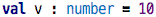src

The thing after the colon is the (explicitly given) type of the value `v`. The literal `10` is the value. We could have written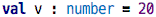src

as well. So both 10 and 20 have type `number`, otherwise we could not legally write this code. The notion of instantiation is actually the inverse relationship of “has type”. So instead of saying that 10 and 20 have type `number`, we could say that these two are both instances of the type `number`. Values are instances of types.

Let us go back to records:src

This example captures the notion of a train connection (guess where I am writing this :-)). We can create values of this type, i.e., record instances by writing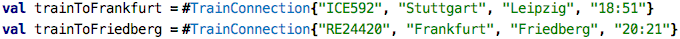src

So what is different here compared to the `number` examples above? We provide values for each of the members of the record. So here, the type can be seen as a kind of template for constructing values. The template defines “holes”, i.e., things that an instance can define freely when they are created. So different instances can be distinguished by the values they have for the “holes”.

The type defines which values are valid for instances. Let’s look at this statement a little bit more closely by looking at the following examples: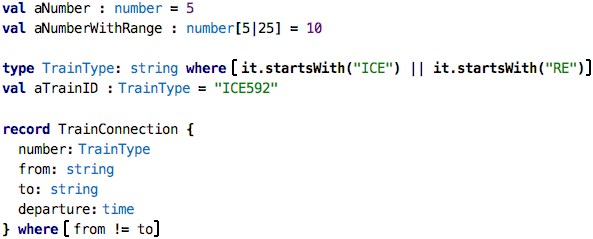src

The first one defines a number without any further constraint (although we know that if we don’t specify a precision, no decimal digits are allowed, so this is a kind of implicit constraint). The `aNumberWithRange` is more picky, because the range specified on the type is of course a constraint on the set of allowed values. The next example defines a new type based on the `string` type. That new type, `TrainType`, has a constraint that expresses that valid values have to begin with the string `ICE` or `RE` (two kinds of trains in Germany, InterCity Express and Regional Express). You can express a constraint on any type using the `where` keyword. For example, you could define the type of odd numbers as `type oddNum : number where isOdd(it)`. Finally, the new version of the `TrainConnection` defines a constraint that expresses that the train cannot arrive in the same city from which it leaves. We get errors when we try to create instances that do not conform to these rules, which is why all the following tests fail.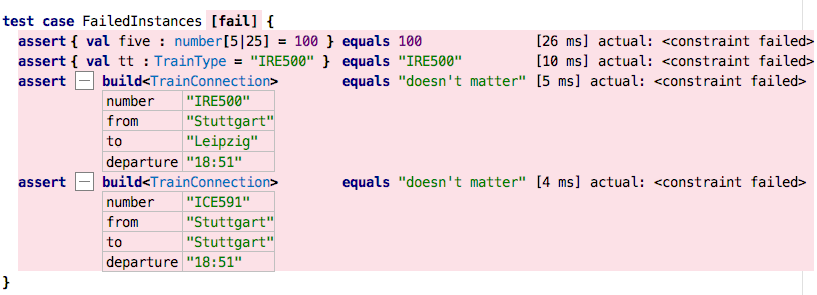src

Failing tests are bad. Always. So, can we write a test that expects the constraints to fail (and then becomes green)? Yes, we can: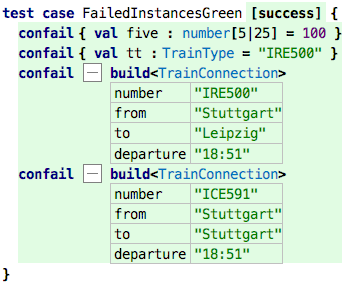src

Constraints expressed this way are checked at runtime, we have to actually run the tests. Checking them in the type system is too complicated. The only exception is the number ranges; here, the IDE actually reports an error as soon as you write the program.

Ok, so this is essentially a recap of what we have seen so far: instances are values that have a particular type, that provide values for the “holes” left open by the type, if any, and respect the constraints expressed by the type, implicitly or explicitly. Let’s look at more examples of this.

### Sheet Templates

Can we play with the idea of instantiation in Spreadsheets? What would this mean? Look at the following example: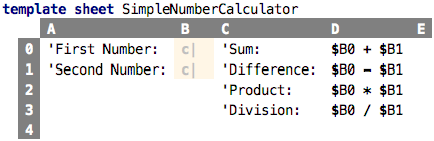src

This sheet computes the sum, difference, product and division of two numbers. The particular numbers for which it does this are not specified. However, this is a template sheet, i.e., a sheet that is intended for instantiation; the two values that are used for the computations are the “holes” in the type, i.e., they are specified in instances. For the expressions in the D column to type check, we have to give a type for the unspecified values in column B. And in fact, the two cells there are constrained, as indicated by the little `c` and the yellowish background color. If we show the sheet in a slightly different way, we can see the constraints: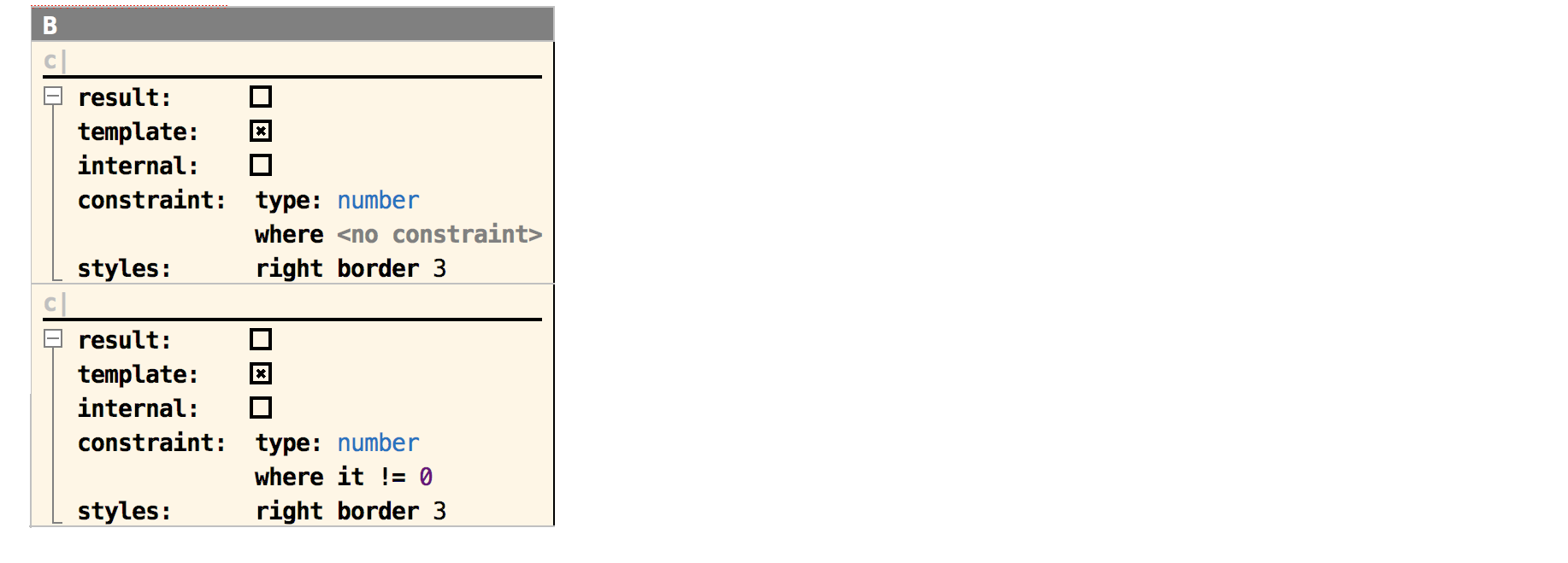We define the type to be `number` for both, and for the second one we even express that the value cannot be zero, because otherwise we’d run into a division by zero when we divide the two numbers in cell D3.

So, let’s create an instance: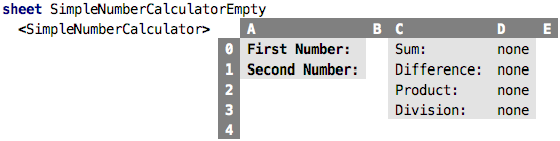src

As you can see, it is automatically shown in “value mode”, where all the expressions are already evaluated. No values have been provided for the two numbers in column B so far. So it is actually an invalid instance. Let’s provide values: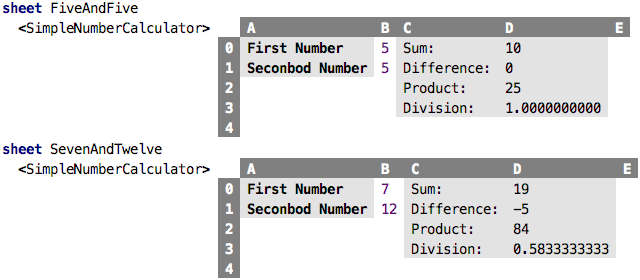src

Why did I show this example? I want to drive home the idea almost anything can be made into a type, and thus be made instantiatable. Just leave holes, let instances fill values for the holes, and check the hole-values provided by the instances against the constraints specified by the type. That’s it!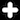Usually an instance retains a reference to its type. In records, notice how an instance `#Person{"Markus", "Voelter"}` references the record `Person`. Similarly, the spreadsheet instances shown above also refer to the template spreadsheet in between the angle brackets. This is so we can check the conformance of the instance against its type.

### Data Flow Blocks

If you are a “business person” you probably find the spreadsheet-based examples intuitive because of your experience with Excel. If you are an engineer, you probably want to see block diagrams. But even if you’re not an engineer, you will learn quite a bit from this example as well. Take a look at the following code: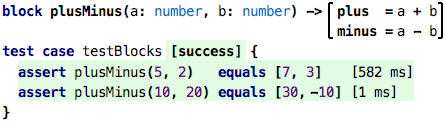src

A block is a new abstraction, you can see it as a function that has more than one result. The `plusMinus` block takes two numbers, and returs two results: the `plus` result where `a` and `b` are added, as well as the `minus` result that subtracts the two. The two results are computed separately, just as if it were a function with two bodies. So nothing really new here. In fact, if you think about it, it’s really almost exactly the same as the instantiatable spreadsheet example: we also calculated several values (sum, difference, product, division) for the same set of inputs.

You can also call a block as if it were a function. However, as we know, functions have only one result. How can we package two results into one? We could use a list, or a record, or something we have not seen before, a tuple: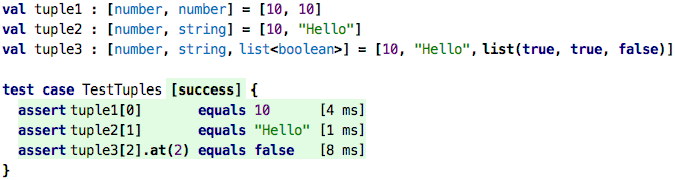src

Tuple types are lists of types enclosed in square brackets, and the values are written using the same notation, of course now as a list of comma-separated expressions. When you have a tuple value in your hand, you can use the `[]` notation to access the tuples’ elements by position.

So, as a brief recap, here’s a comparion between records, lists and tuples:

 Record List Tuple Number of Elements Fixed Variable Fixed Are elements named? Named Anonymous Anonymous All elements same type? Different Types Same Type Different Types Explicitly declared and named? Declared Anonymous Anonymous

So, returning to our blocks, the expression `plusMinus(5, 2)` in the test case calls a block, and receives the two results as a tuple. We express the expected result as a tuple as well. Here is this initial example again:src

Let us define a few more blocks: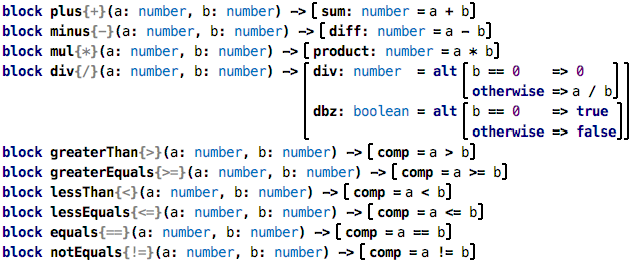src

As you can see, these blocks essentially wrap the basic arithmetic and comparison operators we have used before. Most of them have exactly one output, except the `div` block, which also outputs if a division by zero would have occured because the second argument was zero.

So what is the point of this exercise? These blocks can be used for graphical programming, where we draw diagrams that represent block instances and the flow of data between them. We package such diagrams into yet other blocks, so-called composite blocks, which can then be instantiated further. Let’s illustrate this.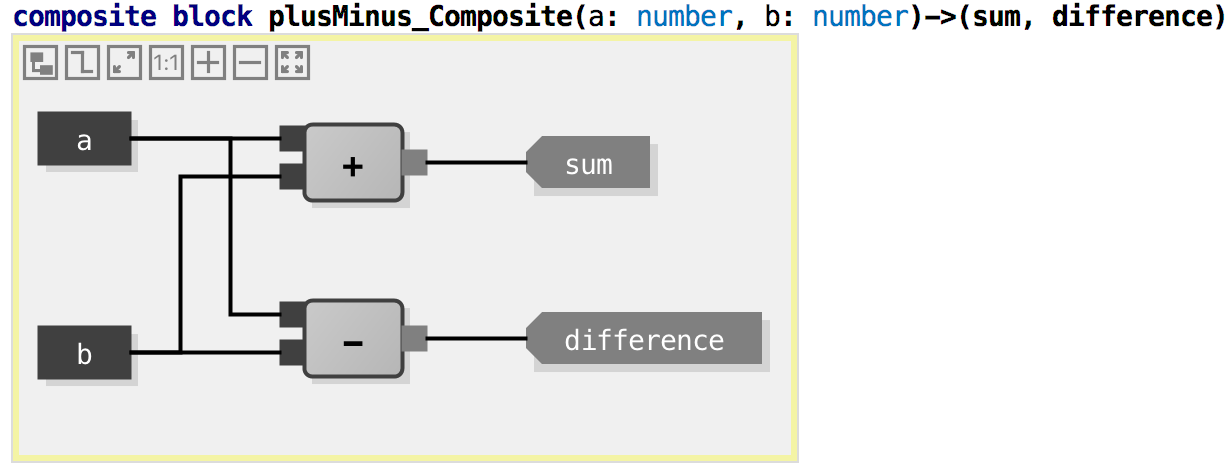This graphical notation works as follows. The dark rectangles on the left (`a`, `b`) are inputs of the current block. The gray boxes with diagonal corners (`sum`, `difference`) are outputs of the block. These are read-only, graphical representations of the block’s interface that is defined textually in the header of the block:The rest of the diagram, i.e., in this example, the `+` and `-` block instances and the connections, are done graphically. You drag and drop blocks from the palette, and then draw the connectors using the mouse: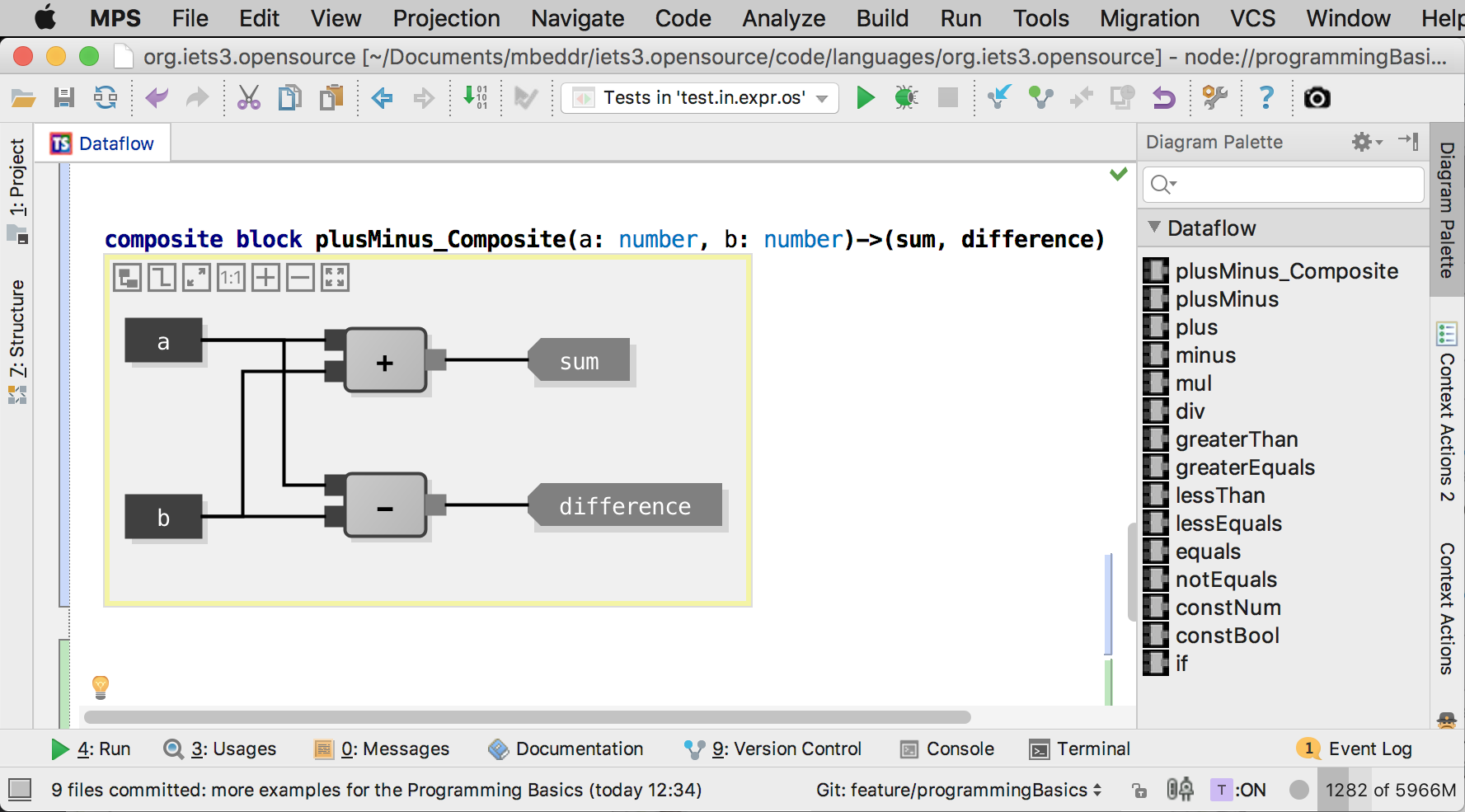So what exactly are we seeing here? The grey rectangles are instances of previously defined blocks; the symbols are defined with the block, see the set of predefined blocks above. The lines represent the data flow. In this example, the data flows from the inputs (`a`, `b`) to the two inputs of the instances inside this composite block. So, as the name suggests, this block computes the sum and difference of the two inputs. Let’s verify the behavior via a few tests: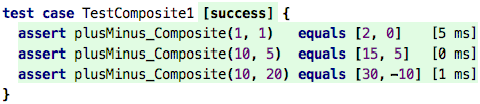src

Let’s make this a little bit more complicated: both the sum and the difference can now be offset by a constant value each. We use the `constNum` block which simply outputs the number that is given to it by the configuration parameter `expr`.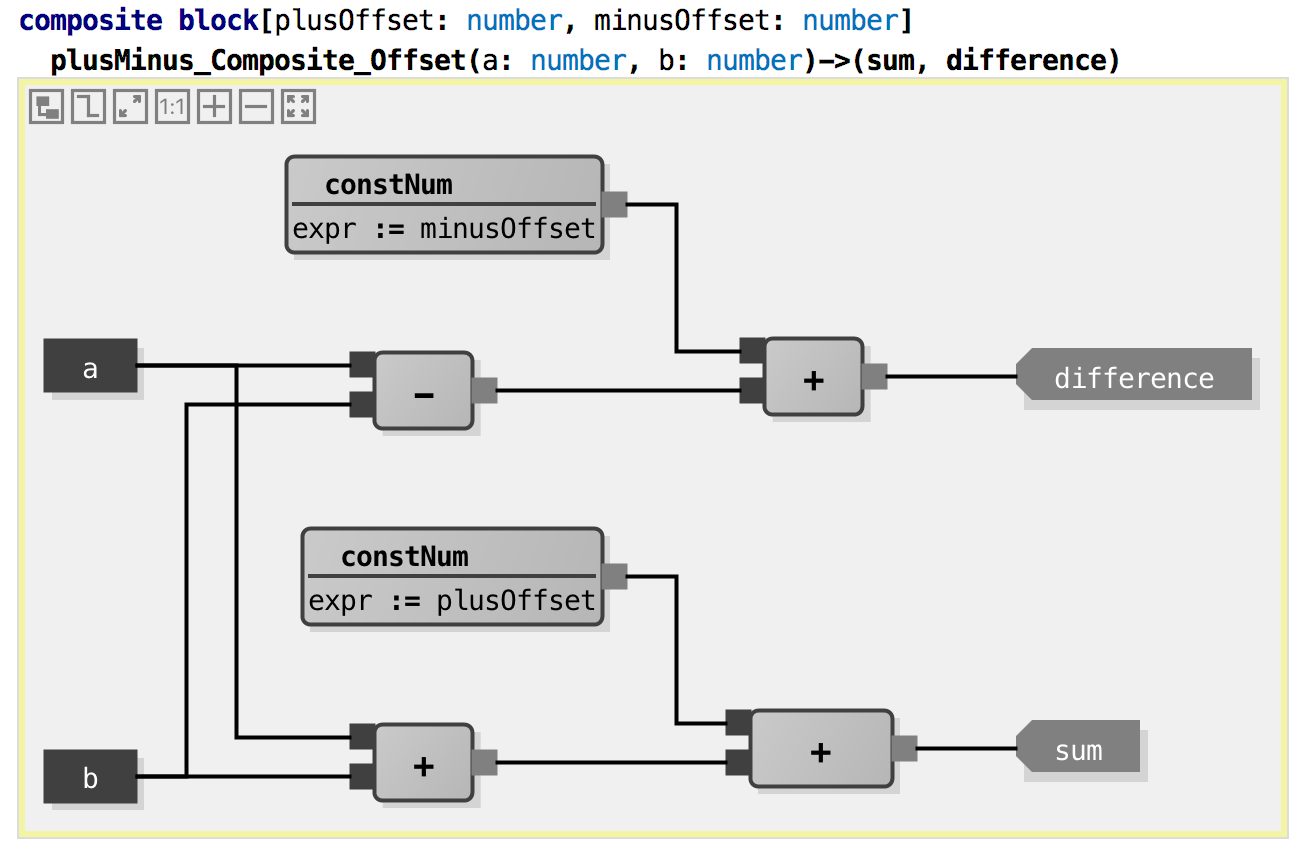Let’s write tests: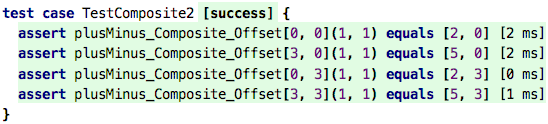src

To return to the discussion about instantiation: this composite block contains several instances of other blocks: 3 of the block `+`, two of the block `constNum`, and one of the block `-`. The composite block can of course also be instantiated: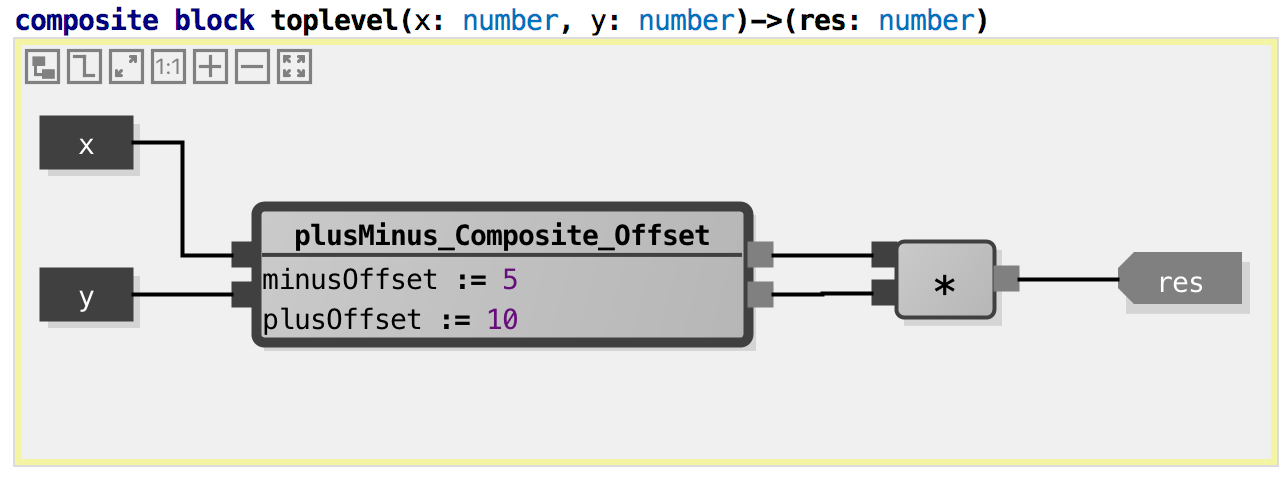Here we have instantiated the composite block from above into a new composite block. Of course, once we do this, we have to specify the two parameter values (`offsetPlus`, `offsetMinus`). To compute one single result, we multiply the sum and the difference. Tests:src

So what is good and bad about this? What is good is that this approach supports nice modularization – by packaging functionality into reusable blocks, we can reuse – reinstantiate – those blocks in different context. Modularization is generally good, and we will discuss it much more in later chapters.

What about the graphical notation? Generally, people tend to like graphical notations: “a picture says more than 1000 words”. However, let’s be realistic: it also takes up a lot of space, and visually following a network of black lines is not necessarily simpler than working with names (if we were to use functions instead of blocks). So how would this same behavior look like if we were to use plain functions? Here is the code if we retain the nesting structure, i.e., each block becomes a function, each instance becomes a function call, and each line becomes a use of a parameter: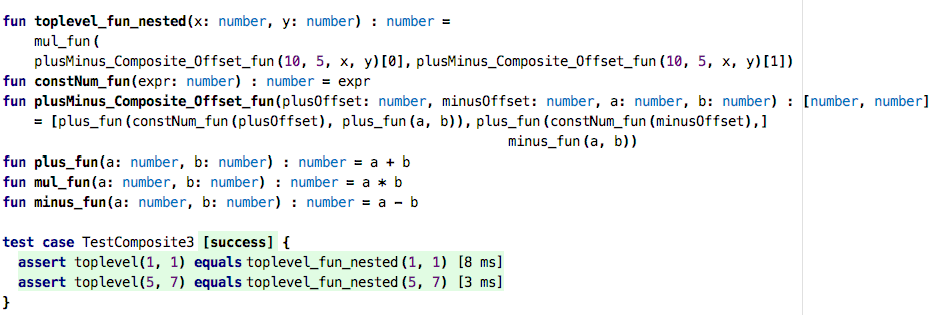src

Admittedly, this does not look much better, in fact, you could justifiable argue that it is less readable. In practice it would be a bit different, since the basic blocks and their functions (for plus, minus, etc.) would be predefined somewhere, so we can mentally remove them. What remains isn’t that bad anymore: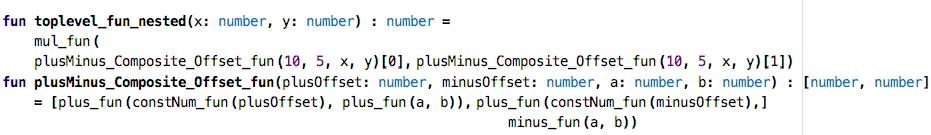src

However, once we inline everything, i.e., we get rid of the extra functions and reduce everything to the bare operators, things look different: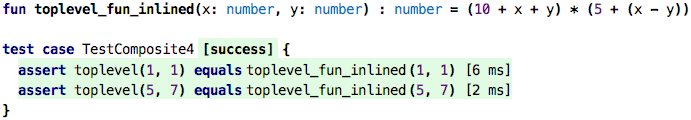src

This is really simple now! So what does this tell us? For fine-grained, basic arithmetics, a graphical notation really isn’t worth it. Once things become more involved, and we maybe even have recursion in the computations (shown as cyclic lines in the diagrams), a graphical notation becomes more interesting. And we also learn that dataflow programming is just another form of functional programming as we have seen it before. No big deal!This style of programming is often used for control systems. There, the semantics of the blocks is a little bit different from the pure functional semantics used here. They might have state, and the system as a whole is also concerned with timing and timed execution of blocks. Again, in such a case, the approach is a little bit more defensible than here. However, even there, the usefulness of the graphical notation is often doubtful.

### Function Values

So, what about functions? Can we instantiate functions? Look at this code: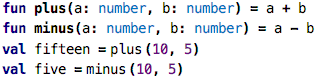src

You could say that the function call “instantiates a function”. After all, the function defines “holes”, i.e., the parameters, and the call provides values. The problem is that the function call executes right away, evaluates to the result value (15 and 5 in the example above), and then assigns that value to the `val`. So the “function instance” created by the call (kind of) has an infinitesimal lifetime, it never really exists. So, can function calls themselves (not the thing they evaluate to) be values? Let’s see.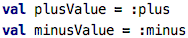src

In this code we create two `val`s, and they represent a value `:plus` and a value `:minus`, respectively. The colon operator here creates a reference to a function. That reference is a value, that value can be passed around and modified, like any other value. What is the type of that value? Let’s ask the tool to automatically annotate the types: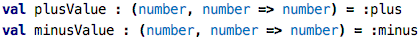src

What we see here are function types, i.e., types that represent functions. The type given here is the type of functions that take two `number`s as arguments and produce another `number`. Both `plus` and `minus` conform to this type; they take two numbers and produce another one.

What can we do with these values? One thing is that we can execute the functions, by passing values: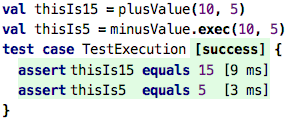src

Executing a function directly is exactly the same as executing a value that contains a reference to that function. You can even use the same `()`-based syntax (as shwon in the `thisIs15` example), alternatively you can also use an explicit call to `exec`.

However, we can do other neat things. For example, as it is typical for instances, we can provide values for parameters, even without executing them at the same time. This is similar to the configuration parameters in the data flow example shown above. Consider the following: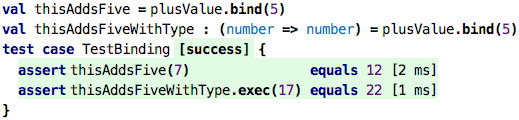src

Using `bind`, you specify some of the parameters of a function call (a process called partial application). So what happens here is that we use the `plusValue` (which is a reference to that original `plus` function that takes two numbers and produces a third one) and bind the first argument to 5. This results in a new function value that now only takes one argument (the first one was just bound to 5) and adds 5 to that argument. We store this new function value in the `val` named `thisAddsFive`. The type, as we see in `thisAddsFiveWithType` is now a function that takes one number and produces another one. As the tests show, we can now use this value as a function with one argument, a function that adds 5 to whatever we pass in.

So, if these things are values, we should be able not just to store them in `val`s, but also to pass them around, right? Absolutely: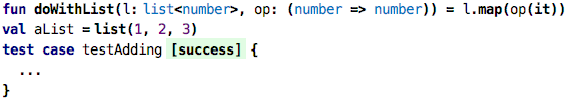src

Check out the function `doWithList`. It takes two arguments. The first one is a regular list of numbers. The second is a function that takes a number and produces a number (like the `thisAddsFive` we just created). The idea of `doWithList` is that is produces a new list, where the function we pass as the second argument is applied to all elements of the list we pass as the first argument. We implement this function using the `map` operator on the list which we have seen before.

So do you realize what we have just done? We have implemented our own version of `map`! We can rename the function and make it an extension function, then we can literally use them in identical ways: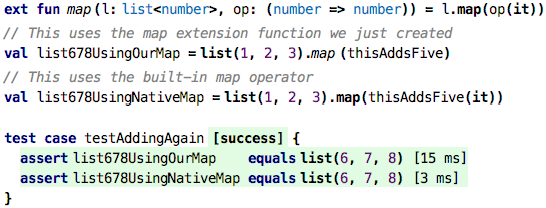src

Well, not quite identical. In the native case we explicitly execute the `thisAddsFive` function value with the `it` that represents the list’s element we currently iterate over. In the case that uses our own `map` extension function we just pass a function value. Can we simplify this?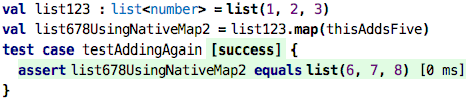src

Indeed we can. So what do these higher-order functions, natively built in (like `map`, `where`, etc.) or built by us exactly do? How do they work? They expect function values, i.e., values of type function type, as arguments. So going back to our own higher-order function …src

… how can we construct valid values for the second argument? Here are three ways: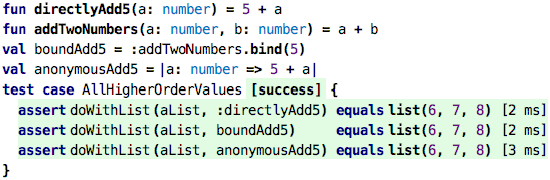src

We can pass a reference to a function whose signature is compatible (first test), we can pass one whose signature has been made compatible through partial application (second test), and we can pass a value that represents an anonymous function. We have not really seen those before, so let’s close with explaining these.

So the `anonymousAdd5` value is what we call a lambda expression, or an anonymous function. It defines one argument, `a: number` and then has a body that adds 5 to that `a`. The lambda itself has no name, but it doesn’t need one, because we assign it to a `val`. The following two are essentially equivalent: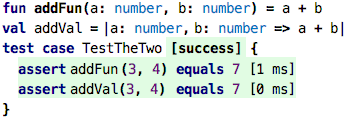src

The latter has the advantage that you can define it also in places other than the top level of a program that contains functions, records or test cases. You can, for example, define it inside a function …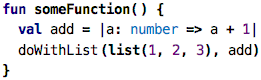src

… or anonynously, in a call: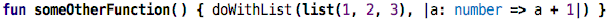src

The `map` on collections is typically used with anonymous function values:src

Because these one-argument anomymous lambdas are extremely common with collections (because one wants to process each element, iteratively), there is a short form for the lambdas that can only be used with these higher-order collection operations. The following code is identical to the previous one: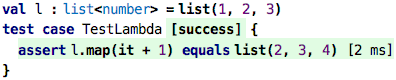src

Here, the argument is automatically named `it`, and it is automatically typed with the element type of the collection on which `map` or `where` is called.

## Wrap up

So this brings us full circle: we have just explained higher-order functions and the lambda expressions we have used before via instantiation. This finishes the second part of the tutorial. Part three, once written, will deal with stateful programs and the passage of time.